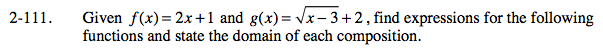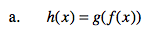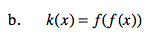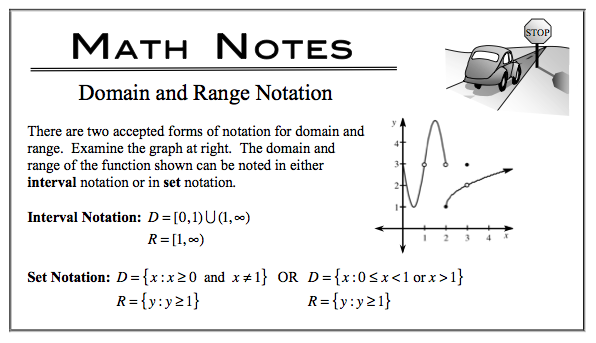### Home > CALC > Chapter 2 > Lesson 2.3.1 > Problem2-111

2-111.
1. Given f(x) = 2x + 1 and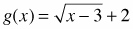, find expressions for the following functions and state the domain of each composition. Homework Help ✎

1. h(x) = g(f(x))

2. k(x) = f(f(x))